# Highest Common Factor

## Notes

### Highest Common Factor:

The Highest Common Factor(HCF) of two or more given numbers is the highest (or greatest) of their common factors. It is also known as Greatest Common Divisor (GCD).

### a) HCF By Prime Factorization Method:

i) Find the HCF of 24 and 32 by the prime factors method.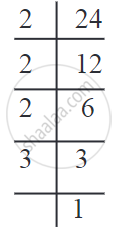24 = 4 × 6

= 2 × 2 × 2 × 3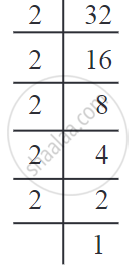32 = 8 × 4

= 2 × 2 × 2 × 2 × 2

The common factor 2 occurs thrice in each number. Therefore, the HCF = 2 × 2 × 2 = 8.

ii) Find the HCF of 195, 312, 546.

 195 = 5 × 39 = 5 × 3 × 13 2 = 4 × 78 = 2 × 2 × 2 × 3 = 2 × 2 × 2 × 3 × 13 6 = 2 × 273 = 2 × 3 × 91 = 2 × 3 × 7 × 13

The common factors 3 and 13 each occur once in all the numbers.
∴ HCF = 3 × 13 = 39

iii) Find the HCF of 60, 12, 36

Let us work out this example in the vertical arrangement. We write all the numbers in one line and find their factors.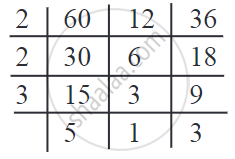∴ HCF = 2 × 2 × 3 = 12
Note that 12 is a divisor of 36 and 60.

Note:

• If one of the given numbers is a divisor of all the others, then it is the HCF of the given numbers.

• If no prime number is a common divisor of all the given numbers, then 1 is their HCF because it is the only common divisor.

### b) The Division Method for Finding the HCF:

i) Find the HCF of 144 and 252.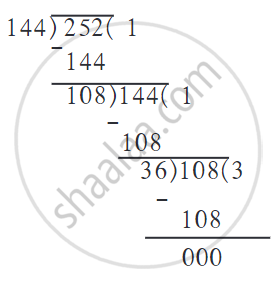1. Divide the bigger number by the smaller one.

2. Divide the previous divisor by the remainder of this division.

3. Divide the divisor of step 2 by the remainder obtained in the division in step 2.

4. Continue like this till the remainder becomes zero. The divisor in the division in which the remainder is zero is the HCF of the given numbers.

∴ The HCF of 144 and 252 = 36.

ii) Reduce (209)/(247) to its simplest form.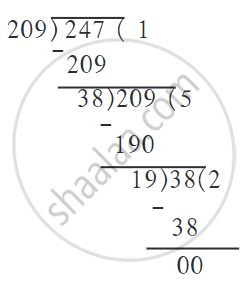To reduce the number to its simplest form, we will find the common factors of 209 and 247.

Let us find their HCF by the division method.

Here, 19 is the HCF. That is, the numerator and denominator are both divisible by 19.

∴ (209)/(247) = (209 ÷ 19)/(247 ÷ 19) = 11/13.

## Example

Find the HCF of the following numbers: 20, 28, and 36.

The HCF of 20, 28 and 36 can also be found by prime factorisation of these numbers as follows: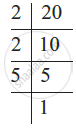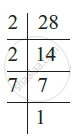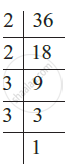Thus,
20 = 2 × 2 × 5
28 = 2 × 2 × 7
36 = 2 × 2 × 3 × 3.

The common factor of 20, 28, and 36 is 2 (occurring twice).

Thus, HCF of 20, 28 and 36 is 2 × 2 = 4.

## Example

Two tankers contain 850 litres and 680 litres of kerosene oil respectively. Find the maximum capacity of a container which can measure the kerosene oil of both the tankers when used an exact number of times.

The required container has to measure both the tankers in a way that a count is an exact number of times. So its capacity must be an exact divisor of the capacities of both the tankers. Moreover, this capacity should be maximum. Thus, the maximum capacity of such a container will be the HCF of 850 and 680.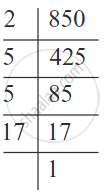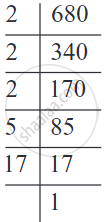Hence,

850 = 2 × 5 × 5 × 17= 2 × 5 × 17 × 5 and

680 = 2 × 2 × 2 × 5 × 17= 2 × 5 × 17 × 2 × 2

The common factors of 850 and 680 are 2, 5 and 17.

Thus, the HCF of 850 and 680 is 2 × 5 × 17 = 170.

Therefore, the maximum capacity of the required container is 170 liters.

It will fill the first container in 5 and the second in 4 refills.

## Example

There are 20 kg of jowar and 50 kg of wheat in a shop. All the grain is to be packed in bags. If all the bags are to have equal weights of grain, what is the maximum weight of grain that can be filled in each bag?

The weight of the grain in each bag must be a factor of 20 and 50.

Besides, the maximum possible weight must be filled in each bag. Hence, let us find the HCF of 20 and 50.

Factors of 20: 1, 2, 4, 5, 10, 20
Factors of 50: 1, 2, 5, 10, 25, 50
Common factors: 1, 2, 5, 10

Of the common factors of 20 and 50, 10 is the greatest, i.e. 10 is the HCF of the numbers 20 and 50.

Therefore, a maximum of 10 kg of grain can be filled in each bag.

## Example

Find the HCF of 10, 15, 12.

10 = 2 × 5
15 = 3 × 5
12 = 2 × 2 × 3
No number except 1 is a common divisor.
Hence, HCF = 1.

## Example

A shop sells a 450 g bottle of jam for 96 rupees and a bigger bottle of 600 g for 124 rupees. Which bottle is it more profitable to buy?

Let us use 150, the HCF of 450 and 600 to compare.

450 = 150 × 3,  600 = 150 × 4

∴ In the small bottle, 150 g of jam costs 96/3 = 32 rupees.

In the large bottle, 150 g of jam costs (124)/4 = 31 rupees.

∴ Thus, it is more profitable to buy the 600 g bottle of jam.

If you would like to contribute notes or other learning material, please submit them using the button below.

### Shaalaa.com

Word Problem On Highest Common Factor [00:21:22]
S
0%